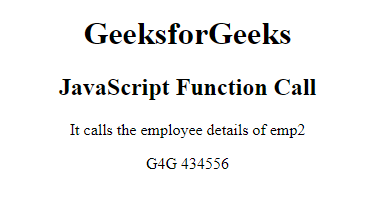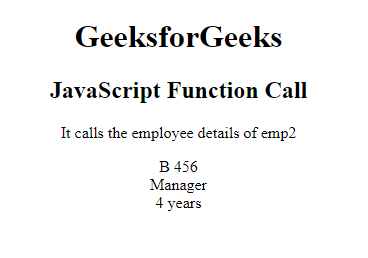# JavaScript Function Call

• Last Updated : 15 Nov, 2021

The Function call is a predefined javascript method, which is used to write methods for different objects. It calls the method, taking the owner object as an argument. The keyword this refers to the “owner” of the function or the object it belongs to. All the functions in javascript are considered as object methods. A function will be the global object if the function is not considered as a method of a JavaScript object.

Syntax:

`call()`

Return Value: It calls and returns a method with the owner object being the argument.

Example 1: This example describes the use of the call() method to call the employee as the argument.

## HTML

 ```<``html``>`` ` `<``head``>``    ``<``title``>JavaScript Function Call```` ` `<``body``>``    ``<``h1``>GeeksforGeeks``    ``<``h2``>JavaScript Function Call``    ``<``p``> It calls the employee details of emp2 `` ` `    ``<``p` `id``=``"GFG"``>`` ` `     ` `    ````    ``<``script``>``    ``var employee = {``        ``details: function() {``            ``return this.name + " " + this.id;``        ``}``    ``}``    ``var emp1 = {``        ``name: "Geeks",``        ``id: "234412",``    ``}``    ``var emp2 = {``        ``name: "G4G",``        ``id: "434556",``    ``}``    ``var x = employee.details.call(emp2);``    ``document.getElementById("GFG").innerHTML = x;``    `````` ` ``

Output:Function Call() Method

Example 2: This example describes the use of function call with arguments.

## HTML

 ```<``html``>`` ` `<``head``>``    ``<``title``> The call() Method with Arguments ```` ` `<``body``>``    ``<``h1``>GeeksforGeeks``    ``<``h2``>JavaScript Function Call``    ``<``p``> It calls the employee details of emp2 `` ` `    ``<``p` `id``=``"GFG"``>`` ` `     ` `    ``<``script``>``    ``var employee = {``        ``details: function(designation, experience) {``            ``return this.name ``            ``+ " " ``            ``+ this.id + "<``br``>" ``            ``+ designation ``            ``+ "<``br``>" ``            ``+ experience;``        ``}``    ``}``    ``var emp1 = {``        ``name: "A",``        ``id: "123",``    ``}``    ``var emp2 = {``        ``name: "B",``        ``id: "456",``    ``}``    ``var x = employee.details.call(emp2, "Manager", "4 years");``    ``document.getElementById("GFG").innerHTML = x;``    `````` ` ``

Output:Function Call() Method with an argument

Supported Browser: